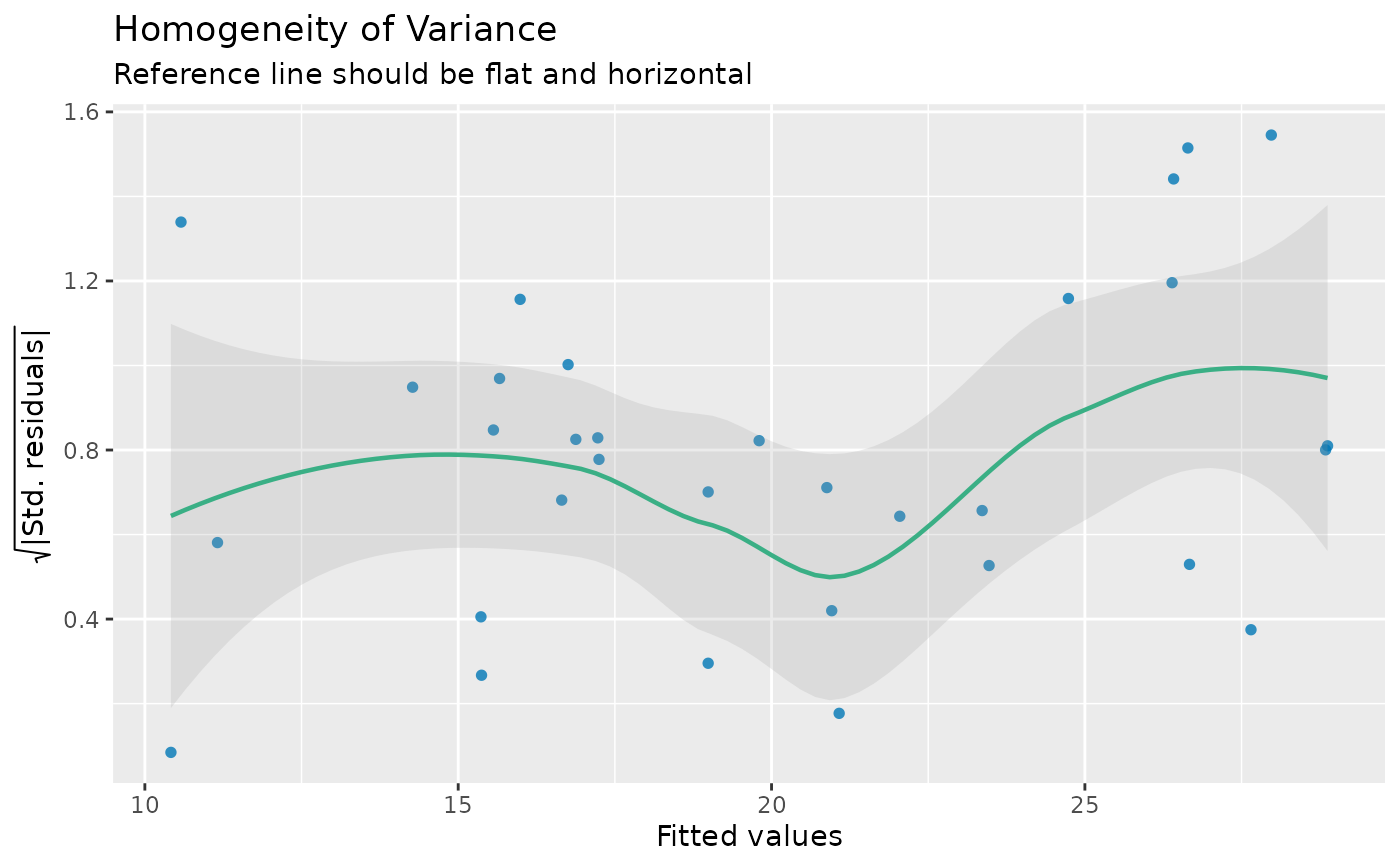Significance testing for linear regression models assumes that the model errors (or residuals) have constant variance. If this assumption is violated the p-values from the model are no longer reliable.

check_heteroscedasticity(x, ...)

check_heteroskedasticity(x, ...)

## Arguments

x A model object. Currently not used.

## Value

Invisibly returns the p-value of the test statistics. A p-value < 0.05 indicates a non-constant variance (heteroskedasticity).

## Details

This test of the hypothesis of (non-)constant error is also called Breusch-Pagan test (1979).

## Note

There is also a plot()-method implemented in the see-package.

Breusch, T. S., and Pagan, A. R. (1979) A simple test for heteroscedasticity and random coefficient variation. Econometrica 47, 1287–1294.

## Examples

m <<- lm(mpg ~ wt + cyl + gear + disp, data = mtcars)
check_heteroscedasticity(m)
#> Warning: Heteroscedasticity (non-constant error variance) detected (p = 0.042).
#>

# plot results
if (require("see")) {
x <- check_heteroscedasticity(m)
plot(x)
}
#> Warning: Heteroscedasticity (non-constant error variance) detected (p = 0.042).
#>